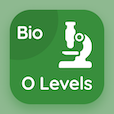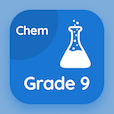Cambridge Online Courses (GCSE)

O Level Physics Quizzes

O Level Physics Quiz PDF - Complete

# Work and Energy Quiz MCQ Online p. 48

Practice Work and Energy quiz questions and answers PDF, work and energy trivia questions 48 to learn online O Level Physics course for online classes. Energy, Work and Power MCQ questions, work and energy Multiple Choice Questions (MCQ) for online college degrees. "Work and Energy Quiz" PDF eBook: acceleration: o level physics, measuring time, pressure physics, density: o level physics, work and energy test prep for ACT subject test tutoring.

"Mass of a car is 500 kg, which is lifted up by a crane at a height of 200 m, the gravity is 10 N kg-1. The Ep of the car will be" MCQ PDF: 10000 j, 1000000 j, 4 j, and 25 j for online colleges for teaching. Solve energy, work and power questions and answers to improve problem solving skills for online bachelor degree programs.

## Trivia Quiz on Work & Energy MCQs

MCQ: Mass of a car is 500 kg, which is lifted up by a crane at a height of 200 m, the gravity is 10 N kg-1. The Ep of the car will be

1000000 J
10000 J
4 J
25 J

MCQ: The volume of mercury is 0.4 m³ and the mass is 5440 kg, the density of mercury is

1360 kg m-3
136 kg m-3
13600 kg m-3
13.6 kg m-3

MCQ: In physics, pressure is defined as

P = A × F
P = A/F
P = F/A
P = F/d

MCQ: The error due to manually stopping a stopwatch is termed as

climax error
human reaction error
human reaction time
ratability error

MCQ: A bus travelling eastwards at 60 m s-1 takes 10s to come to halt, its deceleration is equal to

6 m s-1 eastward
-6 m s-1 eastward
6 m s-1² eastward
-6 m s-1² eastward

### More Quizzes from O Level Physics Course